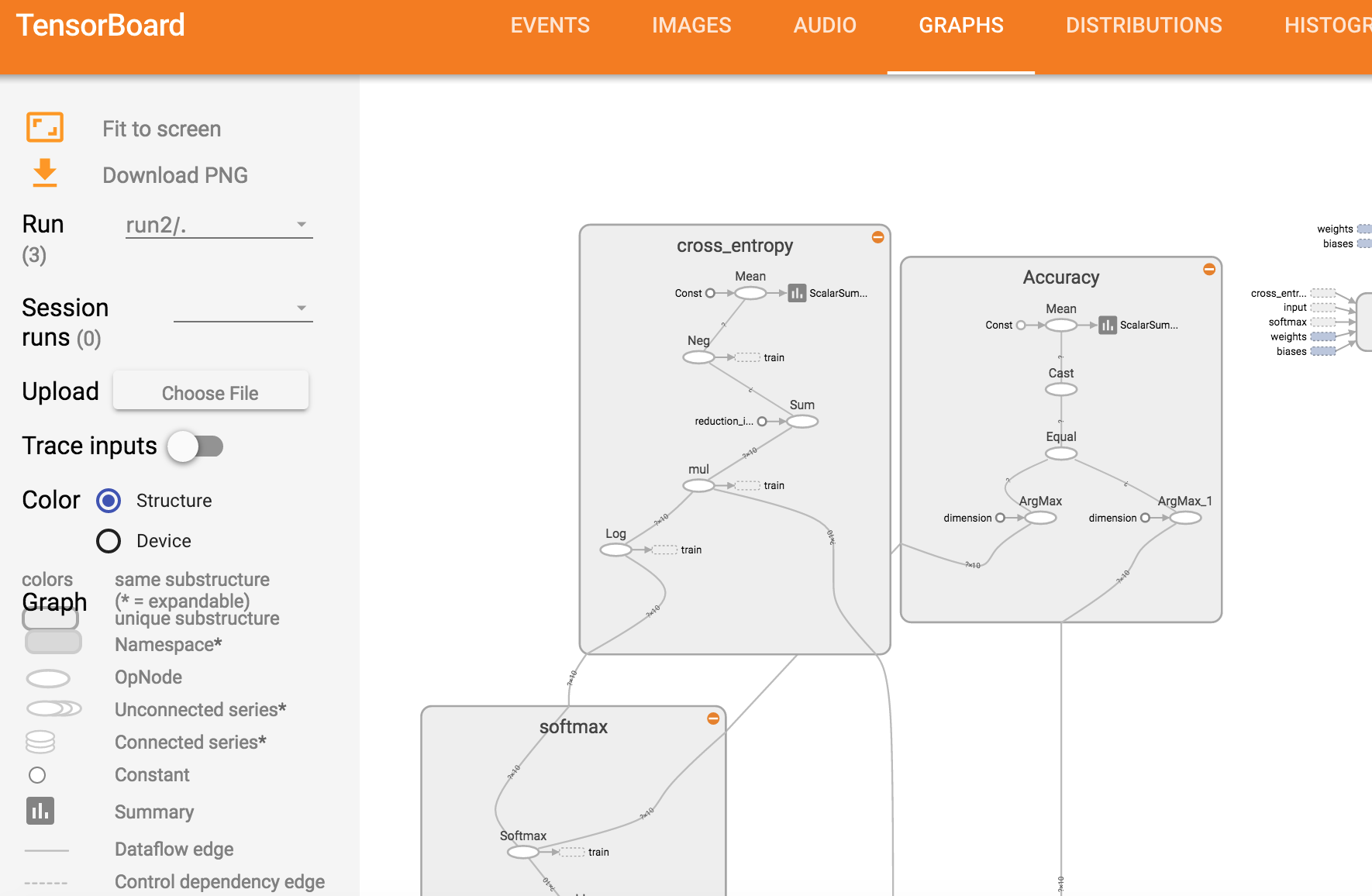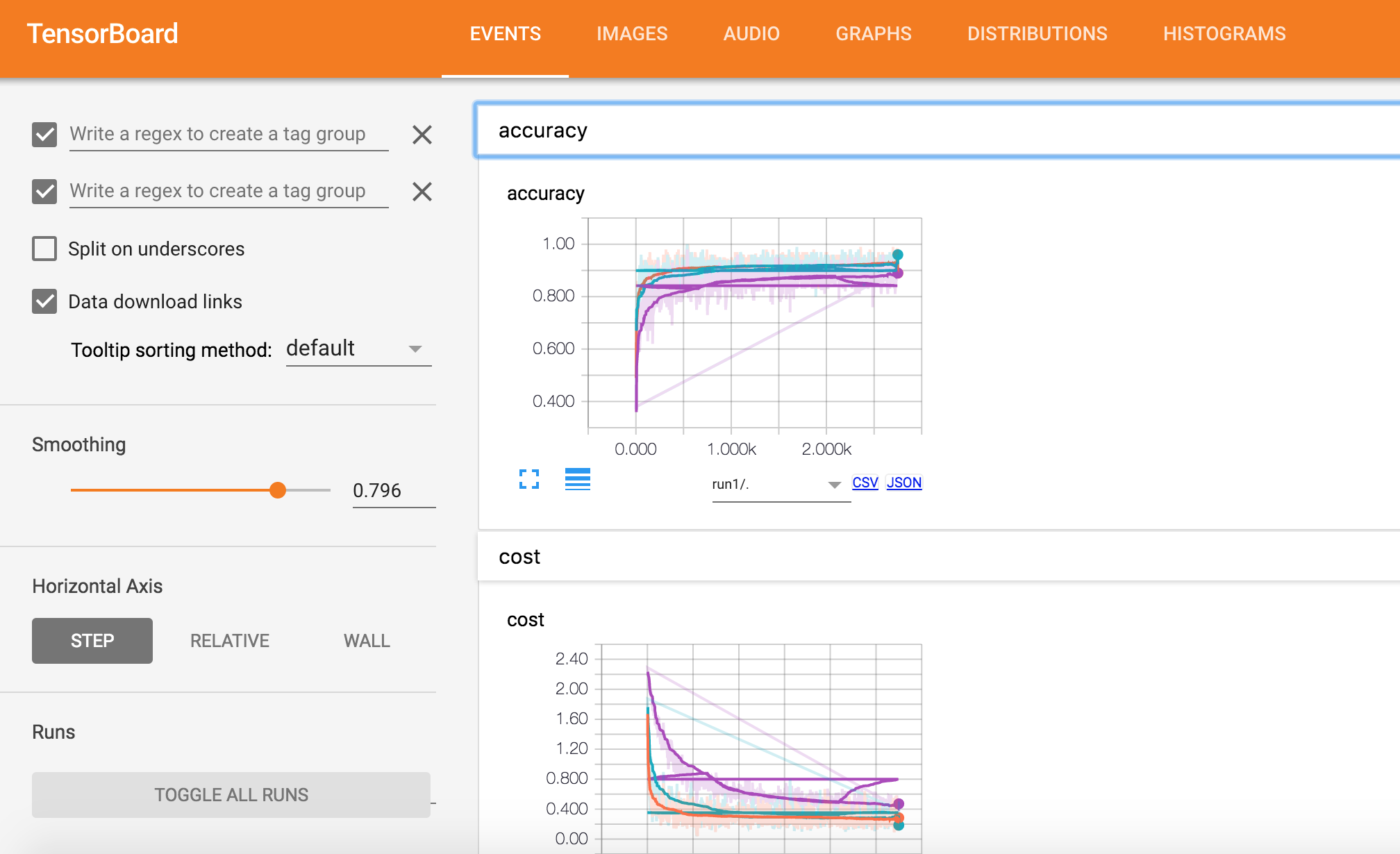# 如何使用 TensorFlow Tensorboard 观察训练，调节参数

A brief and concise tutorial on how to visualize different aspects such as the loss of your neural network using tensorboard.

# TL;DR

A brief and concise tutorial on how to visualize different aspects such as the loss of your neural network using tensorboard.

# Context

We are going to work with a fully-connected neural network using the MNIST dataset. I’m going to use the network I have introduced in an earlier post. It achieves on the test-set an accuracy of ~90%. This is not bad but we have no clue what is actually going on or how our model looks like. In this post we will add the necessary commands to visualize the graph and some training values using tensorboard. If you haven’t installed tensorflow yet look at this post. You also want to have a look at the official tensorflow documentation.

## Write a Log File and Run Tensorboard

Tensorflow summaries are essentially logs. And in order to write logs we need a log writer (or what it is called in tensorflow) a SummaryWriter. So for starters, we’ll add the following line before our train loop.

writer = tf.train.SummaryWriter(logs_path, graph=tf.get_default_graph())



This will create a log folder and save the graph structure. We can now start tensorboard.

tensorboard --logdir=run1:/tmp/tensorflow/ --port 6006A messy graph since we have not annotated anything.
You will notice that tensorboard is not able to find scalar values as we have not specifically logged any. You can, however, look at the tensorflow graph in the Graph tab but the visualization of our not annotated graph looks messy. To clean up the visualization of our model in tensorboard we need to add the scope of our variables and a name for our placeholders and variables. So we add with statements and name parameters like in the following example. You can find the complete code at the end of this article.

# input images

with tf.name_scope('input'):
# None -> batch size can be any size, 784 -> flattened mnist image
x = tf.placeholder(tf.float32, shape=[None, 784], name="x-input")
# target 10 output classes
y_ = tf.placeholder(tf.float32, shape=[None, 10], name="y-input")


I’ve had trouble reloading the graphs in tensorboard (tensorflow release 0.8.0) after rerunning my script in jupyter. To get a correct graph representation you should stop tensorboard and jupyter, delete your tensorflow logdir, restart jupyter, run the script, and then restart tensorboard. After those steps the graph visualization should render correctly. We are not logging any scalar values yet but as you see below our graph is now much cleaner and easier to read.The graph visualization I got for the current example.

## Log Dynamic Values

So far all our changes are only modifying how our graph looks in tensorboard. In this second part, we are now going to add summaries to log specific variables like the training error of our model. To log specific scalar values we need to create operations which we can then execute in our session. Such an operation can be created with the scalar_summary() function. You can find other summary operators here.

### create a summary for our cost and accuracy

tf.scalar_summary("cost", cross_entropy)
tf.scalar_summary("accuracy", accuracy)


Instead of executing every summary operation individually we can merge them all together into a single merged summary operation.

summary_op = tf.merge_all_summaries()


We can then execute this operation together with our train operation inside our train cycle and write the values into our log file using the SummaryWriter object we created earlier.

### perform the operations we defined earlier on batch

, summary = sess.run([train_op, summary_op], feed_dict={x: batch_x, y: batch_y})

### write log

writer.add_summary(summary, epoch * batch_count + i)


You can now start tensorboard and see a cost and accuracy graph in the Events tab. I you want to display several runs in your graph you need to run your script several times and save the log files into seperate folders. You can then start tensorboard reading from several folders.tensorboard --logdir=run1:/tmp/mnist/1,run2:/tmp/mnist/2 --port=6006


A graph showing the cost progres for two different runs.
Here you have to complete code of this example.

# More creditsChatopera博客 聊天机器人 机器学习 智能客服01-0854
11-102124
10-07437
01-1530
01-0886
01-0799
01-0275
01-0272
12-29603
12-293839
12-2656
12-25237
12-2563
12-01154
11-30264
11-12263
11-07562
11-03371
11-03159
10-20436